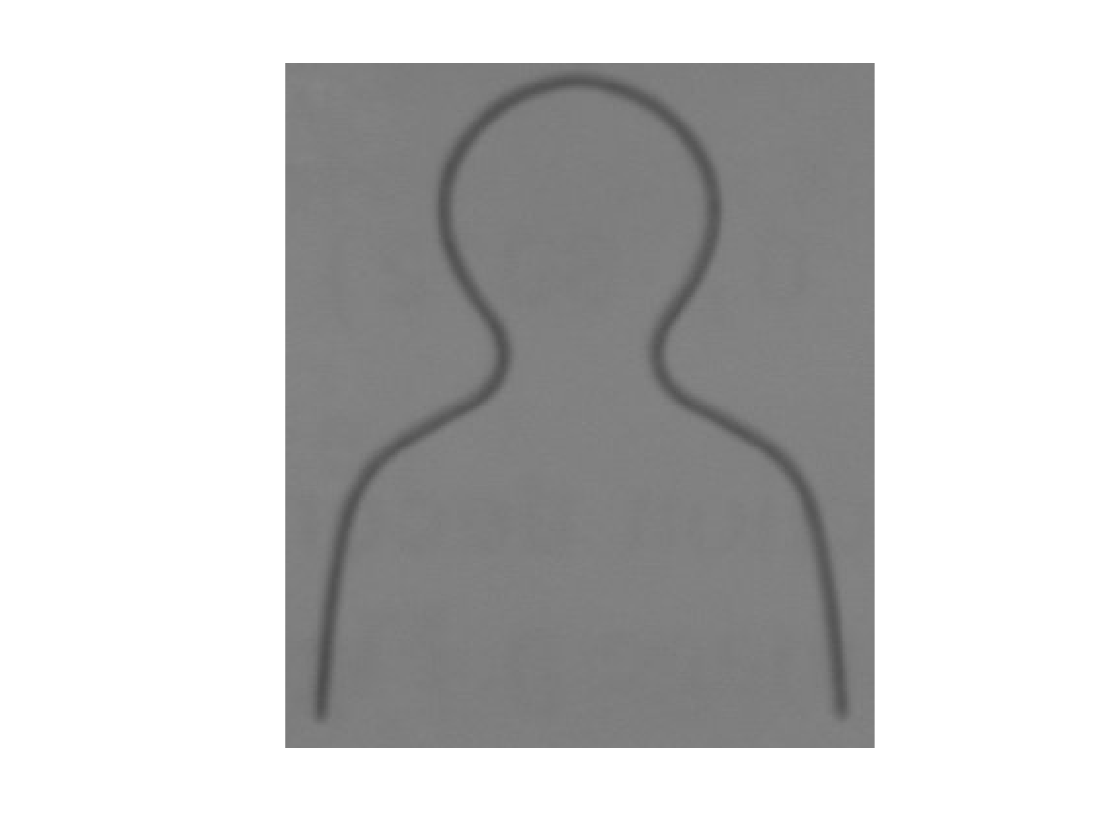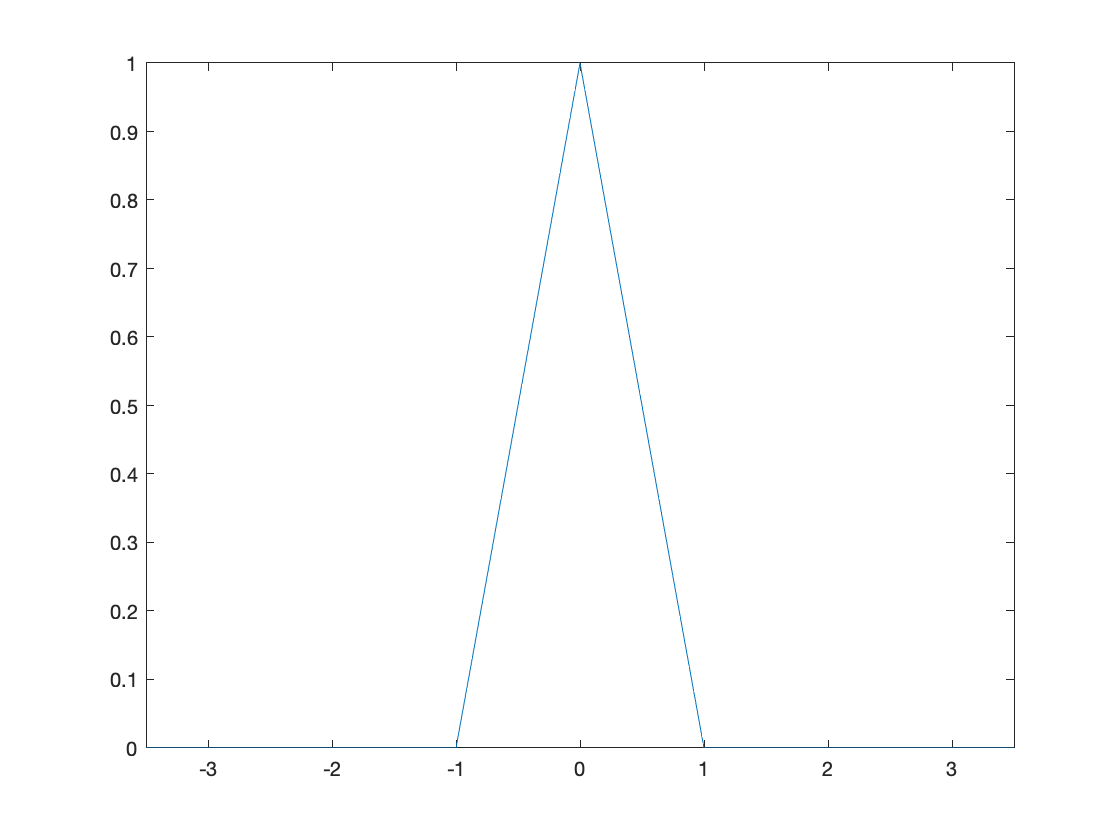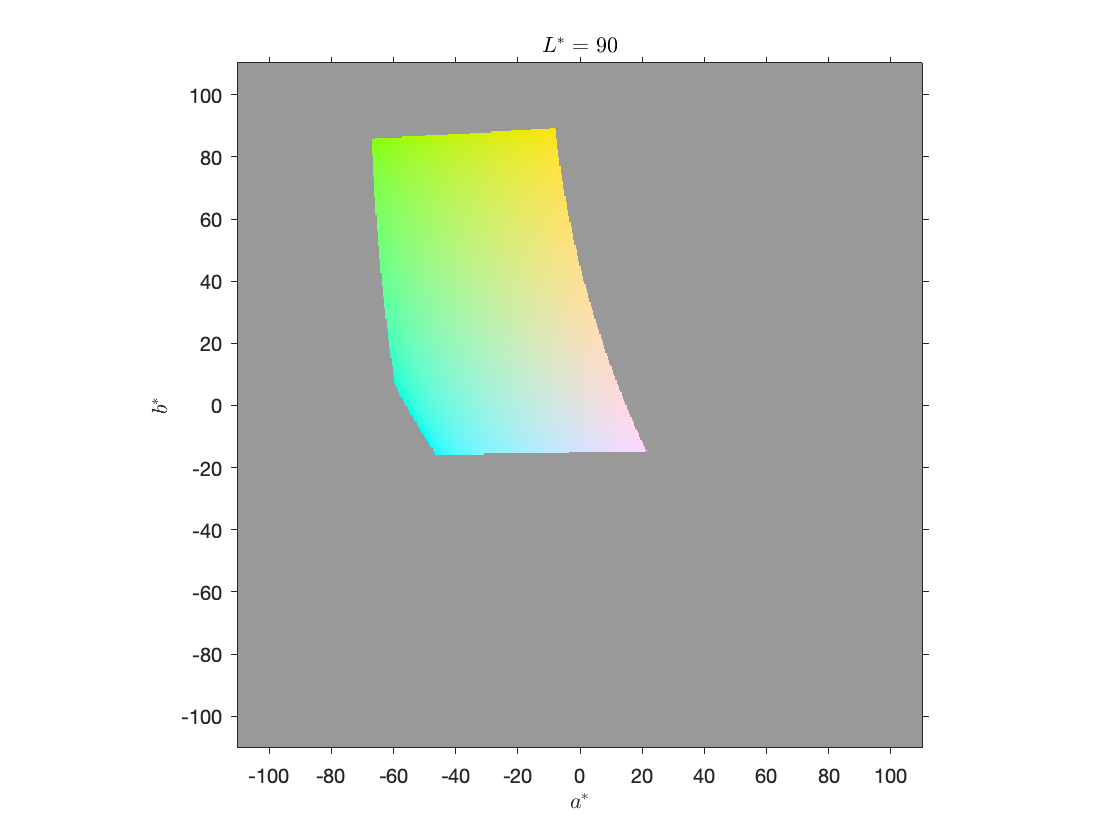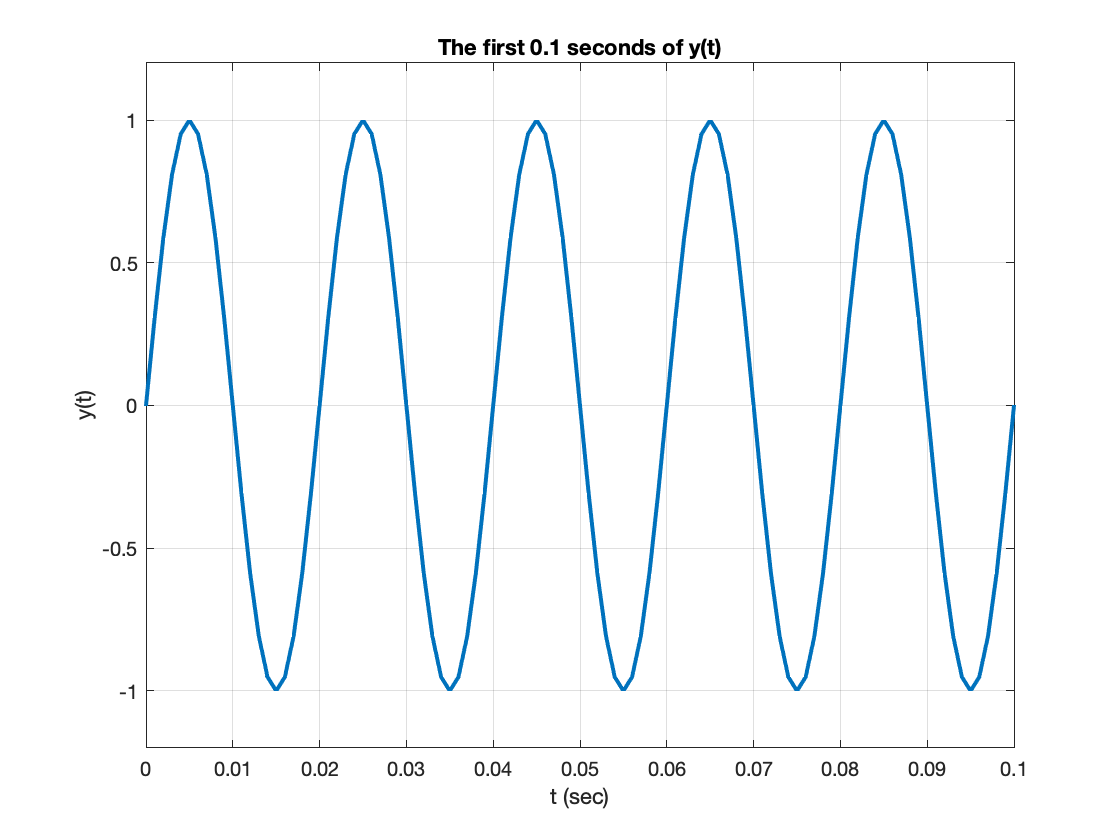Community Profile# Steve Eddins

### MathWorks

Last seen: 12日 前 2006 以来アクティブ

MATLAB user since 1987. MathWorker since 1993. Amateur French horn player. MATLAB design and development, image processing algorithms.

Image processing blog: https://blogs.mathworks.com/steve/

#### Statistics

All
••••••••••••バッジを表示

#### Content Feed

Can a MATLAB function edit the input?
You'll need your class to subclass handle. See the documentation page "Implementing Linked Lists with Classes" for details.

2ヶ月 前 | 0

| 採用済み

Matlab working very slow on my m1 MacBook Pro

4ヶ月 前 | 2

Shouldn't bwconvhull() be idempotent?
Hi Matt, At a general level - and speaking from too much personal experience - expectations about geometrical operations such a...

4ヶ月 前 | 2

| 採用済み

Issue in plotting binary image boundaries with real axis values
The function bwboundaries returns boundary values in pixel coordinates. You'll need to scale those to world coordinates yourself...

4ヶ月 前 | 0

| 採用済み

How to change boundary width in Active Contour Method?
Call visboundaries with an output argument. That output argument will be an hggroup object containing two lines. You can then ma...

4ヶ月 前 | 0

| 採用済み

Use imbinarize to Threshold Gray-Scale Images
Today, I want to convince you to use imbinarize instead of im2bw.Background: I recently saw some data suggesting that many...

4ヶ月 前NaN Sightings
Some of us at MathWorks like to share our "NaN sightings." Here is an example to show what I mean. This is a screenshot of...

5ヶ月 前How to Use a Custom Interpolation Kernel with imresize
Recently, I was talking with MathWorks writer Jessica Bernier about the reference page for the imresize function. Jessica...

6ヶ月 前How to Run Blog Post Code in Your Browser
I have published more than 560 blog posts here since 2006, and I estimate that about 98% of them started out as MATLAB...

6ヶ月 前Plotting a* and b* colors
Today's blog post comes from planning one topic, but then taking a sharp left turn and doing something else completely. I...

6ヶ月 前Having graycomatrix rescaling problem
Here is the doc for the NumLevels parameter: Number of gray levels, specified as an integer. For example, if NumLevels is 8, gr...

7ヶ月 前 | 0

| 採用済み

How to find the center position under the saturation spot signal
Try converting the image to binary using imbinarize and then using regionprops to compute the centroid. rgb = imread('image.bmp...

7ヶ月 前 | 0

| 採用済み

How to bold x axis labels
Try setting the TickLabelsInterpreter property to 'tex', like this: plot(1:10) ax = gca; ax.XAxis.TickLabelInterpreter = 'tex...

7ヶ月 前 | 0

three difference vectors and plots
I have copy-pasted your code below, exactly as entered in your question. It runs and produces the plot with no error. I suggest ...

7ヶ月 前 | 2

Problem with datetime and daily values
Use a timetable and the retime function. >> T = readtable("data.xlsx"); >> head(T) ans = 8×5 table Date ...

7ヶ月 前 | 0

| 採用済み

What is the best way to count the occurrences of two table columns together?
In my previous answer, I forgot that grpstats is in the Statistics and Machine Learning Toolbox. Here is an answer using groupsu...

7ヶ月 前 | 1

What is the best way to count the occurrences of two table columns together?
I think grpstats will do what you want: >> grpstats(T,["A" "B"]) ans = 4×3 table A B Grou...

7ヶ月 前 | 0

Find peaks not working on my data set
I suspect the issue is with the x-coordinates that you are using to plot the peaks. You are plotting the peaks against the indic...

8ヶ月 前 | 1

| 採用済み

Assign values in a matrix
One idea is to use a timetable and the retime function. Suppose I have the following matrix for a 4-minute charge and a 3-minute...

8ヶ月 前 | 0

| 採用済み

Code is showing Undefined function 'pixelHull' for input arguments of type 'double'.
I wrote a function called pixelHull as part of my 16-Mar-2018 blog post. You can find the function listing near the bottom of th...

8ヶ月 前 | 0

| 採用済み

FFT Spectral Leakage
A MATLAB user recently contacted MathWorks tech support to ask why the output of fft did not meet their expectations, and...

8ヶ月 前Binarize image for specific colour
Use the Color Thresholder app to generate the code needed to do this segmentation. See my 14-Oct-2019 and 25-Apr-2014 blog posts...

9ヶ月 前 | 0

Plotting signal of a region of interest
A possible explanation is that you have a variable called "size" or "mean" in your workspace. For example, if you have a variabl...

9ヶ月 前 | 0

How to apply a threshold value to the cell array
I think writing a for-loop would be most straightforward way to go. Something like this: for k = 1:numel(C) C_k = C{k}; ...

9ヶ月 前 | 1

| 採用済み

How to change the font of a pie chart?
The text labels created by the pie function are not controlled by the font properties of the containing axes object. They are in...

9ヶ月 前 | 2

| 採用済み

superimpose matrices (n,n,m) to (n,n,1)
Here are some options. Compute and plot the maximum value at each pixel: imagesc(max(a100,[],3)) Compute and plot the minimum...

10ヶ月 前 | 0

| 採用済み

Image conversion from uint16 to uint8 mismatch
im2uint8 is using a scale factor of (255/65535), not 1/256. Note that scaling the maximum of the uint16 range, 65535, by 1/256 ...

10ヶ月 前 | 1

| 採用済み

How do I map array values on a logical array
Use logical indexing. C = zeros(size(B)); C(logical(B)) = A

10ヶ月 前 | 1

| 採用済み

Create a 7x7 convolution kernel which has an equivalent effect to three passes with a 3x3 mean filter.
Convolution is associative: So, your 7x7 convolution kernel would be the convolution of the mean filters: Use the 'full' o...

10ヶ月 前 | 0

pgm image lossless compression
Write the image to a PNG file. The PNG format uses lossless compression. imwrite(A,'myfile.png') Read it back in using imread....

10ヶ月 前 | 0